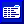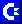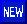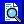# Hexed By Numbers

J. Blake Lambert, Assistant Editor

Reading and writing bytes, and directly modifying disk tracks and sectors is something like PEEKing and POKEing memory locations. You are advancing beyond simple BASIC programs into the realm of machine language. Sooner or later, you face the challenge of learning new, more convenient, numbering systems. Since computers only understand binary (base two) numbers and most people understand decimal (base ten) numbers, it's advantageous to learn how to translate from one to the other. To understand these number systems, let's think a minute about our normal counting method.

Our decimal (base ten) system evolved simply because humans have ten fingers. When we count in decimal, we start with zero and add ones until we get to nine, at which point we're out of numbers. To get to ten, we place a zero in the "ones" column and add a new column to the left, called the "tens" column. The next column to the left is named "hundreds," which is ten times ten (or 10^2),

Binary counting is the same, but we run out of digits faster, because the computer has only two "fingers." That is, the electronic "switches" with which it counts are either off (0) or on (1). We count zero then one, and that's it. We start a new column called "twos," place a one in it, and clear the "ones" column, The next number is 11 and we're out of digits again. The "fours" column is created (two times two, or 2^2), and we have the number 100, then 101, 110, 111 and 1000 (which equals eight in decimal, 2^3). The problem with binary is that it takes too much room to write even small numbers. For example, the decimal number 255 written in binary is 11111111.

It requires 16 ones and zeros to describe a memory location on a VIC or 64. (Decimal 49152 translates to the unwieldy binary number 1100000000000000.) This is why hexadecimal, called hex by most computerists, is useful.

Hex is a simple shorthand for binary, but it requires learning a few new numbers. Just as the decimal number system has ten digits available (0 through 9), hex requires 16. These 16 digits are 0 through 9, then A, 8, C, D, E, F, After counting to \$F (the dollar sign represents "hex," the F stands for decimal 15), we next count \$10, by placing a one in the "sixteens" column. The hex number \$FF in decimal, then, equals 15 times 16, plus 15. Conveniently, this turns out to be decimal 255, so the eight-column binary representation (1111 1111) can be written using just two hex numerals (\$FF). It's much easier to remember shorter numbers, so hex is usually preferred.

Here's a counting table that should help you understand how the systems work.

Binary Decimal Hexadecimal
0 0 \$0
1 1 \$1
10 2 \$2
11 3 \$3
100 4 \$4
101 5 \$5
110 6 \$6
111 7 \$7
1000 8 \$8
1001 9 \$9
1010 10 \$A
1011 11 \$B
1100 12 \$C
1101 13 \$D
1110 14 \$E
1111 15 \$F
10000 16 \$10

Published in COMPUTE!'s Gazette, Issue 15, September 1984. Copyright © 1984 COMPUTE! Publications, Inc.This page has been created by Sami Rautiainen. Read the small print. Last updated September 05, 2020.